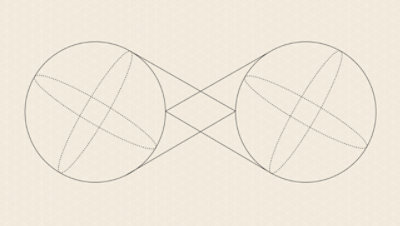# What is quantum computing?

It's the use of quantum mechanics to run calculations on specialized hardware.

## Introduction to quantum computing

To fully define quantum computing, we need to define some key terms first.

### What is quantum?

The quantum in "quantum computing" refers to the quantum mechanics that the system uses to calculate outputs. In physics, a quantum is the smallest possible discrete unit of any physical property. It usually refers to properties of atomic or subatomic particles, such as electrons, neutrinos, and photons.

### What is a qubit?

A qubit is the basic unit of information in quantum computing. Qubits play a similar role in quantum computing as bits play in classical computing, but they behave very differently. Classical bits are binary and can hold only a position of 0 or 1, but qubits can hold a superposition of all possible states.

### What is quantum computing?

Quantum computers harness the unique behavior of quantum physics—such as superposition, entanglement, and quantum interference—and apply it to computing. This introduces new concepts to traditional programming methods.

## Superposition

In superposition, quantum particles are a combination of all possible states. They fluctuate until they're observed and measured. One way to picture the difference between binary position and superposition is to imagine a coin. Classical bits are measured by "flipping the coin" and getting heads or tails. However, if you were able to look at a coin and see both heads and tails at the same time, as well as every state in between, the coin would be in superposition.

## Entanglement

Entanglement is the ability of quantum particles to correlate their measurement results with each other. When qubits are entangled, they form a single system and influence each other. We can use the measurements from one qubit to draw conclusions about the others. By adding and entangling more qubits in a system, quantum computers can calculate exponentially more information and solve more complicated problems.## Quantum interference

Quantum interference is the intrinsic behavior of a qubit, due to superposition, to influence the probability of it collapsing one way or another. Quantum computers are designed and built to reduce interference as much as possible and ensure the most accurate results. To this end, Microsoft uses topological qubits, which are stabilized by manipulating their structure and surrounding them with chemical compounds that protect them from outside interference.

## How does quantum computing work?

A quantum computer has three primary parts:

• An area that houses the qubits
• A method for transferring signals to the qubits
• A classical computer to run a program and send instructions

For some methods of qubit storage, the unit that houses the qubits is kept at a temperature just above absolute zero to maximize their coherence and reduce interference. Other types of qubit housing use a vacuum chamber to help minimize vibrations and stabilize the qubits.

Signals can be sent to the qubits using a variety of methods, including microwaves, laser, and voltage.

## Quantum computer uses and application areas

A quantum computer can't do everything faster than a classical computer, but there are a few areas where quantum computers have the potential to make a big impact.

### Quantum simulation

Quantum computers work exceptionally well for modeling other quantum systems because they use quantum phenomena in their computation. This means that they can handle the complexity and ambiguity of systems that would overload classical computers. Examples of quantum systems that we can model include photosynthesis, superconductivity, and complex molecular formations.

### Cryptography

Classical cryptography—such as the Rivest–Shamir–Adleman (RSA) algorithm that’s widely used to secure data transmission—relies on the intractability of problems such as integer factorization or discrete logarithms. Many of these problems can be solved more efficiently using quantum computers.

### Optimization

Optimization is the process of finding the best solution to a problem given its desired outcome and constraints. In science and industry, critical decisions are made based on factors such as cost, quality, and production time—all of which can be optimized. By running quantum-inspired optimization algorithms on classical computers, we can find solutions that were previously impossible. This helps us find better ways to manage complex systems such as traffic flows, airplane gate assignments, package deliveries, and energy storage.

### Quantum machine learning

Machine learning on classical computers is revolutionizing the world of science and business. However, training machine learning models comes with a high computational cost, and that has hindered the scope and development of the field. To speed up progress in this area, we're exploring ways to devise and implement quantum software that enables faster machine learning.

### Search

A quantum algorithm developed in 1996 dramatically sped up the solution to unstructured data searches, running the search in fewer steps than any classical algorithm could.

## Azure Quantum resources

Build quantum solutions today as an early adopter of Azure Quantum Preview, a full-stack open cloud ecosystem. Access software, hardware, and pre-built solutions and start developing on a trusted, scalable, and secure platform.